# Tag: topology

A few MathOverflow threads I bookmarked in 2010 for various reasons.

This is a belated response to a Math-Overflow exchange between Thomas Riepe and Chandan Singh Dalawat asking for a possible connection between Connes’ noncommutative geometry approach to the Riemann hypothesis and the Langlands program.

Here’s the punchline : a large chunk of the Connes-Marcolli book Noncommutative Geometry, Quantum Fields and Motives can be read as an exploration of the noncommutative boundary to the Langlands program (at least for $GL_1$ and $GL_2$ over the rationals $\mathbb{Q}$).

Recall that Langlands for $GL_1$ over the rationals is the correspondence, given by the Artin reciprocity law, between on the one hand the abelianized absolute Galois group

$Gal(\overline{\mathbb{Q}}/\mathbb{Q})^{ab} = Gal(\mathbb{Q}(\mu_{\infty})/\mathbb{Q}) \simeq \hat{\mathbb{Z}}^*$

and on the other hand the connected components of the idele classes

$\mathbb{A}^{\ast}_{\mathbb{Q}}/\mathbb{Q}^{\ast} = \mathbb{R}^{\ast}_{+} \times \hat{\mathbb{Z}}^{\ast}$

The locally compact Abelian group of idele classes can be viewed as the nice locus of the horrible quotient space of adele classes $\mathbb{A}_{\mathbb{Q}}/\mathbb{Q}^{\ast}$. There is a well-defined map

$\mathbb{A}_{\mathbb{Q}}’/\mathbb{Q}^{\ast} \rightarrow \mathbb{R}_{+} \qquad (x_{\infty},x_2,x_3,\ldots) \mapsto | x_{\infty} | \prod | x_p |_p$

from the subset $\mathbb{A}_{\mathbb{Q}}’$ consisting of adeles of which almost all terms belong to $\mathbb{Z}_p^{\ast}$. The inverse image of this map over $\mathbb{R}_+^{\ast}$ are precisely the idele classes $\mathbb{A}^{\ast}_{\mathbb{Q}}/\mathbb{Q}^{\ast}$. In this way one can view the adele classes as a closure, or ‘compactification’, of the idele classes.

This is somewhat reminiscent of extending the nice action of the modular group on the upper-half plane to its badly behaved action on the boundary as in the Manin-Marcolli cave post.

The topological properties of the fiber over zero, and indeed of the total space of adele classes, are horrible in the sense that the discrete group $\mathbb{Q}^*$ acts ergodically on it, due to the irrationality of $log(p_1)/log(p_2)$ for primes $p_i$. All this is explained well (in the semi-local case, that is using $\mathbb{A}_Q’$ above) in the Connes-Marcolli book (section 2.7).

In much the same spirit as non-free actions of reductive groups on algebraic varieties are best handled using stacks, such ergodic actions are best handled by the tools of noncommutative geometry. That is, one tries to get at the geometry of $\mathbb{A}_{\mathbb{Q}}/\mathbb{Q}^{\ast}$ by studying an associated non-commutative algebra, the skew-ring extension of the group-ring of the adeles by the action of $\mathbb{Q}^*$ on it. This algebra is known to be Morita equivalent to the Bost-Connes algebra which is the algebra featuring in Connes’ approach to the Riemann hypothesis.

It shouldn’t thus come as a major surprise that one is able to recover the other side of the Langlands correspondence, that is the Galois group $Gal(\mathbb{Q}(\mu_{\infty})/\mathbb{Q})$, from the Bost-Connes algebra as the symmetries of certain states.

In a similar vein one can read the Connes-Marcolli $GL_2$-system (section 3.7 of their book) as an exploration of the noncommutative closure of the Langlands-space $GL_2(\mathbb{A}_{\mathbb{Q}})/GL_2(\mathbb{Q})$.

At the moment I’m running a master-seminar noncommutative geometry trying to explain this connection in detail. But, we’re still in the early phases, struggling with the topology of ideles and adeles, reciprocity laws, L-functions and the lot. Still, if someone is interested I might attempt to post some lecture notes here.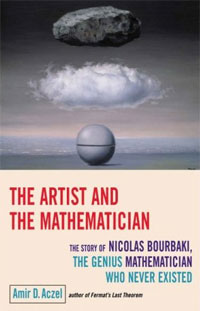Over the week-end I read The artist and the mathematician (subtitle : The story of Nicolas Bourbaki, the genius mathematician who never existed) by Amir D. Aczel.

Whereas the central character of the book should be Bourbaki, it focusses more on two of Bourbaki’s most colorful members, André Weil and Alexander Grothendieck, and the many stories and myths surrounding them.

The opening chapter (‘The Disappearance’) describes the Grothendieck’s early years (based on the excellent paper by Allyn Jackson Comme Appelé du Néant ) and his disappearance in the Pyrenees in the final years of last century. The next chapter (‘An Arrest in Finland’) recount the pre-WW2 years of Weil and the myth of his arrest in Finland and his near escape from execution (based on Weil’s memoires The Apprenticeship of a Mathematician). Chapter seven (‘The Café’) describes the first 10 proto-Bourbaki meetings following closely the study ‘A Parisian Café and Ten Proto-Bourbaki Meetings (1934-1935)‘ by Liliane Beaulieu. Etc. etc.

All the good ‘Bourbaki’-stories get a place in this book, not always historically correct. For example, on page 90 it is suggested that all of the following jokes were pulled at the Besse-conference, July 1935 : the baptizing of Nicolas, the writing of the Comptes-Rendus paper, the invention of the Bourbaki-daughter Betti and the printing of the wedding invitation card. In reality, all of these date from much later, the first two from the autumn of 1935, the final two no sooner than april 1939…

One thing I like about this book is the connection it makes with other disciplines, showing the influence of Bourbaki’s insistence on ‘structuralism’ in fields as different as philosophy, linguistics, anthropology and literary criticism. One example being Weil’s group-theoretic solution to the marriage-rules problem in tribes of Australian aborigines studied by Claude Lévi-Strauss, another the literary group Oulipo copying Bourbaki’s work-method.

Another interesting part is Aczel’s analysis of Bourbaki’s end. In the late 50ties, Grothendieck tried to convince his fellow Bourbakis to redo their work on the foundations of mathematics, changing these from set theory to category theory. He failed as others felt that the foundations had already been laid and there was no going back. Grothendieck left, and Bourbaki would gradually decline following its refusal to accept new methods. In Grothendieck’s own words (in “Promenade” 63, n. 78, as translated by Aczel) :

“Additionally, since the 1950s, the idea of structure has become passé, superseded by the influx of new ‘categorical’ methods in certain of the most dynamical areas of mathematics, such as topology or algebraic geometry. (Thus, the notion of ‘topos’ refuses to enter into the ‘Bourbaki sack’ os structures, decidedly already too full!) In making this decision, in full cognizance, not to engage in this revision, Bourbaki has itself renounced its initial ambition, which has been to furnish both the foundations and the basic language for all of modern mathematics.”

Finally, it is interesting to watch Aczel’s own transformation throughout the book, from slavishly copying the existing Weil-myths and pranks at the beginning of the book, to the following harsh criticism on Weil, towards the end (p. 209) :

“From other information in his autobiography, one gets the distinct impression that Weil was infatuated with the childish pranks of ‘inventing’ a person who never existed, creating for him false papers and a false identity, complete with a daughter, Betti, who even gets married, parents and relatives, and membership in a nonexistent Academy of Sciences of the nonexistent nation of Polvedia (sic).
Weil was so taken with these activities that he even listed, as his only honor by the time of his death ‘Member, Poldevian Academy of Sciences’. It seems that Weil could simply not go beyond these games: he could not grasp the deep significance and power of the organization he helped found. He was too close, and thus unable to see the great achievements Bourbaki was producing and to acknowledge and promote these achievements. Bourbaki changed the way we do mathematics, but Weil really saw only the pranks and the creation of a nonexistent person.”

Judging from my own reluctance to continue with the series on the Bourbaki code, an overdose reading about Weil’s life appears to have this effect on people…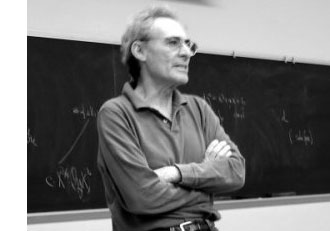In the previous posts, we have depicted the ‘arithmetic line’, that is the prime numbers, as a ‘line’ and individual primes as ‘points’.

However, sometime in the roaring 60-ties, Barry Mazur launched the crazy idea of viewing the affine spectrum of the integers, $\mathbf{spec}(\mathbb{Z})$, as a 3-dimensional manifold and prime numbers themselves as knots in this 3-manifold…

After a long silence, this idea was taken up recently by Mikhail Kapranov and Alexander Reznikov (1960-2003) in a talk at the MPI-Bonn in august 1996. Pieter Moree tells the story in his recollections about Alexander (Sacha) Reznikov in Sipping Tea with Sacha : “Sasha’s paper is closely related to his paper where the analogy of covers of three-manifolds and class field theory plays a big role (an analogy that was apparently first noticed by B. Mazur). Sasha and Mikhail Kapranov (at the time also at the institute) were both very interested in this analogy. Eventually, in August 1996, Kapranov and Reznikov both lectured on this (and I explained in about 10 minutes my contribution to Reznikov’s proof). I was pleased to learn some time ago that this lecture series even made it into the literature, see Morishita’s ‘On certain analogies between knots and primes’ J. reine angew. Math 550 (2002) 141-167.”

Here’s a part of what is now called the Kapranov-Reznikov-Mazur dictionary :What is the rationale behind this dictionary? Well, it all has to do with trying to make sense of the (algebraic) fundamental group $\pi_1^{alg}(X)$ of a general scheme $X$. Recall that for a manifold $M$ there are two different ways to define its fundamental group $\pi_1(M)$ : either as the closed loops in a given basepoint upto homotopy or as the automorphism group of the universal cover $\tilde{M}$ of $M$.

For an arbitrary scheme the first definition doesn’t make sense but we can use the second one as we have a good notion of a (finite) cover : an etale morphism $Y \rightarrow X$ of the scheme $X$. As they form an inverse system, we can take their finite automorphism groups $Aut_X(Y)$ and take their projective limit along the system and call this the algebraic fundamental group $\pi^{alg}_1(X)$.

Hendrik Lenstra has written beautiful course notes on ‘Galois theory for schemes’ on all of this starting from scratch. Besides, there are also two video-lectures available on this at the MSRI-website : Etale fundamental groups 1 by H.W. Lenstra and Etale fundamental groups 2 by F. Pop.

But, what is the connection with the ‘usual’ fundamental group in case both of them can be defined? Well, by construction the algebraic fundamental group is always a profinite group and in the case of manifolds it coincides with the profinite completion of the standard fundamental group, that is,
$\pi^{alg}_1(M) \simeq \widehat{\pi_1(M)}$ (recall that the cofinite completion is the projective limit of all finite group quotients).

Right, so all we have to do to find a topological equivalent of an algebraic scheme is to compute its algebraic fundamental group and find an existing topological space of which the profinite completion of its standard fundamental group coincides with our algebraic fundamental group. An example : a prime number $p$ (as a ‘point’ in $\mathbf{spec}(\mathbb{Z})$) is the closed subscheme $\mathbf{spec}(\mathbb{F}_p)$ corresponding to the finite field $\mathbb{F}_p = \mathbb{Z}/p\mathbb{Z}$. For any affine scheme of a field $K$, the algebraic fundamental group coincides with the absolute Galois group $Gal(\overline{K}/K)$. In the case of $\mathbb{F}_p$ we all know that this abslute Galois group is isomorphic with the profinite integers $\hat{\mathbb{Z}}$. Now, what is the first topological space coming to mind having the integers as its fundamental group? Right, the circle $S^1$. Hence, in arithmetic topology we view prime numbers as topological circles, that is, as knots in some bigger space.

But then, what is this bigger space? That is, what is the topological equivalent of $\mathbf{spec}(\mathbb{Z})$? For this we have to go back to Mazur’s original paper Notes on etale cohomology of number fields in which he gives an Artin-Verdier type duality theorem for the affine spectrum $X=\mathbf{spec}(D)$ of the ring of integers $D$ in a number field. More precisely, there is a non-degenerate pairing $H^r_{et}(X,F) \times Ext^{3-r}_X(F, \mathbb{G}_m) \rightarrow H^3_{et}(X,F) \simeq \mathbb{Q}/\mathbb{Z}$ for any constructible abelian sheaf $F$. This may not tell you much, but it is a ‘sort of’ Poincare-duality result one would have for a compact three dimensional manifold.

Ok, so in particular $\mathbf{spec}(\mathbb{Z})$ should be thought of as a 3-dimensional compact manifold, but which one? For this we have to compute the algebraic fundamental group. Fortunately, this group is trivial as there are no (non-split) etale covers of $\mathbf{spec}(\mathbb{Z})$, so the corresponding 3-manifold should be simple connected… but wenow know that this has to imply that the manifold must be $S^3$, the 3-sphere! Summarizing : in arithmetic topology, prime numbers are knots in the 3-sphere!

More generally (by the same arguments) the affine spectrum $\mathbf{spec}(D)$ of a ring of integers can be thought of as corresponding to a closed oriented 3-dimensional manifold $M$ (which is a cover of $S^3$) and a prime ideal $\mathfrak{p} \triangleleft D$ corresponds to a knot in $M$.

But then, what is an ideal $\mathfrak{a} \triangleleft D$? Well, we have unique factorization of ideals in $D$, that is, $\mathfrak{a} = \mathfrak{p}_1^{n_1} \ldots \mathfrak{p}_k^{n_k}$ and therefore $\mathfrak{a}$ corresponds to a link in $M$ of which the constituent knots are the ones corresponding to the prime ideals $\mathfrak{p}_i$.

And we can go on like this. What should be an element $w \in D$? Well, it will be an embedded surface $S \rightarrow M$, possibly with a boundary, the boundary being the link corresponding to the ideal $\mathfrak{a} = Dw$ and Seifert’s algorithm tells us how we can produce surfaces having any prescribed link as its boundary. But then, in particular, a unit $w \in D^*$ should correspond to a closed surface in $M$.

And all these analogies carry much further : for example the class group of the ring of integers $Cl(D)$ then corresponds to the torsion part $H_1(M,\mathbb{Z})_{tor}$ because principal ideals $Dw$ are trivial in the class group, just as boundaries of surfaces $\partial S$ vanish in $H_1(M,\mathbb{Z})$. Similarly, one may identify the unit group $D^*$ with $H_2(M,\mathbb{Z})$… and so on, and on, and on…

More links to papers on arithmetic topology can be found in John Baez’ week 257 or via here.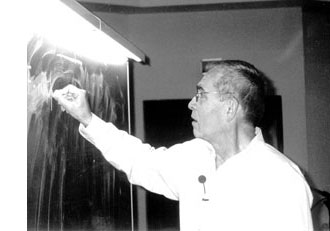Mumford’s drawing has a clear emphasis on the vertical direction. The set of all vertical lines corresponds to taking the fibers of the natural ‘structural morphism’ : $\pi~:~\mathbf{spec}(\mathbb{Z}[t]) \rightarrow \mathbf{spec}(\mathbb{Z})$ coming from the inclusion $\mathbb{Z} \subset \mathbb{Z}[t]$. That is, we consider the intersection $P \cap \mathbb{Z}$ of a prime ideal $P \subset \mathbb{Z}[t]$ with the subring of constants.

Two options arise : either $P \cap \mathbb{Z} \not= 0$, in which case the intersection is a principal prime ideal $~(p)$ for some prime number $p$ (and hence $P$ itself is bigger or equal to $p\mathbb{Z}[t]$ whence its geometric object is contained in the vertical line $\mathbb{V}((p))$, the fiber $\pi^{-1}((p))$ of the structural morphism over $~(p)$), or, the intersection $P \cap \mathbb{Z}[t] = 0$ reduces to the zero ideal (in which case the extended prime ideal $P \mathbb{Q}[x] = (q(x))$ is a principal ideal of the rational polynomial algebra $\mathbb{Q}[x]$, and hence the geometric object corresponding to $P$ is a horizontal curve in Mumford’s drawing, or is the whole arithmetic plane itself if $P=0$).

Because we know already that any ‘point’ in Mumford’s drawing corresponds to a maximal ideal of the form $\mathfrak{m}=(p,f(x))$ (see last time), we see that every point lies on precisely one of the set of all vertical coordinate axes corresponding to the prime numbers ${~\mathbb{V}((p)) = \mathbf{spec}(\mathbb{F}_p[x]) = \pi^{-1}((p))~}$. In particular, two different vertical lines do not intersect (or, in ringtheoretic lingo, the ‘vertical’ prime ideals $p\mathbb{Z}[x]$ and $q\mathbb{Z}[x]$ are comaximal for different prime numbers $p \not= q$).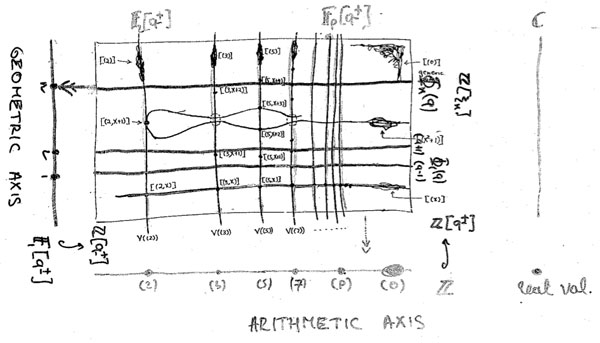That is, the structural morphism is a projection onto the “arithmetic axis” (which is $\mathbf{spec}(\mathbb{Z})$) and we get the above picture. The extra vertical line to the right of the picture is there because in arithmetic geometry it is customary to include also the archimedean valuations and hence to consider the ‘compactification’ of the arithmetic axis $\mathbf{spec}(\mathbb{Z})$ which is $\overline{\mathbf{spec}(\mathbb{Z})} = \mathbf{spec}(\mathbb{Z}) \cup { v_{\mathbb{R}} }$.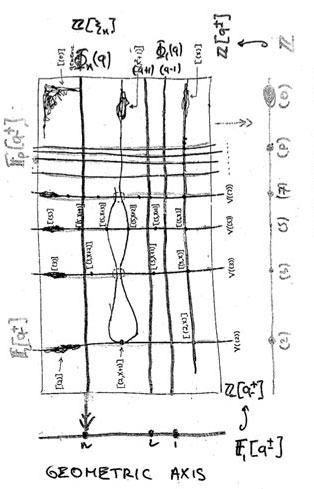Yuri I. Manin is advocating for years the point that we should take the terminology ‘arithmetic surface’ for $\mathbf{spec}(\mathbb{Z}[x])$ a lot more seriously. That is, there ought to be, apart from the projection onto the ‘z-axis’ (that is, the arithmetic axis $\mathbf{spec}(\mathbb{Z})$) also a projection onto the ‘x-axis’ which he calls the ‘geometric axis’.

But then, what are the ‘points’ of this geometric axis and what are their fibers under this second projection?

We have seen above that the vertical coordinate line over the prime number $~(p)$ coincides with $\mathbf{spec}(\mathbb{F}_p[x])$, the affine line over the finite field $\mathbb{F}_p$. But all of these different lines, for varying primes $p$, should project down onto the same geometric axis. Manin’s idea was to take therefore as the geometric axis the affine line $\mathbf{spec}(\mathbb{F}_1[x])$, over the virtual field with one element, which should be thought of as being the limit of the finite fields $\mathbb{F}_p$ when $p$ goes to one!

How many points does $\mathbf{spec}(\mathbb{F}_1[x])$ have? Over a virtual object one can postulate whatever one wants and hope for an a posteriori explanation. $\mathbb{F}_1$-gurus tell us that there should be exactly one point of size n on the affine line over $\mathbb{F}_1$, corresponding to the unique degree n field extension $\mathbb{F}_{1^n}$. However, it is difficult to explain this from the limiting perspective…

Over a genuine finite field $\mathbb{F}_p$, the number of points of thickness $n$ (that is, those for which the residue field is isomorphic to the degree n extension $\mathbb{F}_{p^n}$) is equal to the number of monic irreducible polynomials of degree n over $\mathbb{F}_p$. This number is known to be $\frac{1}{n} \sum_{d | n} \mu(\frac{n}{d}) p^d$ where $\mu(k)$ is the Moebius function. But then, the limiting number should be $\frac{1}{n} \sum_{d | n} \mu(\frac{n}{d}) = \delta_{n1}$, that is, there can only be one point of size one…

Alternatively, one might consider the zeta function counting the number $N_n$ of ideals having a quotient consisting of precisely $p^n$ elements. Then, we have for genuine finite fields $\mathbb{F}_p$ that $\zeta(\mathbb{F}_p[x]) = \sum_{n=0}^{\infty} N_n t^n = 1 + p t + p^2 t^2 + p^3 t^3 + \ldots$, whence in the limit it should become
$1+t+t^2 +t^3 + \ldots$ and there is exactly one ideal in $\mathbb{F}_1[x]$ having a quotient of cardinality n and one argues that this unique quotient should be the unique point with residue field $\mathbb{F}_{1^n}$ (though it might make more sense to view this as the unique n-fold extension of the unique size-one point $\mathbb{F}_1$ corresponding to the quotient $\mathbb{F}_1[x]/(x^n)$…)

A perhaps more convincing reasoning goes as follows. If $\overline{\mathbb{F}_p}$ is an algebraic closure of the finite field $\mathbb{F}_p$, then the points of the affine line over $\overline{\mathbb{F}_p}$ are in one-to-one correspondence with the maximal ideals of $\overline{\mathbb{F}_p}[x]$ which are all of the form $~(x-\lambda)$ for $\lambda \in \overline{\mathbb{F}_p}$. Hence, we get the points of the affine line over the basefield $\mathbb{F}_p$ as the orbits of points over the algebraic closure under the action of the Galois group $Gal(\overline{\mathbb{F}_p}/\mathbb{F}_p)$.

‘Common wisdom’ has it that one should identify the algebraic closure of the field with one element $\overline{\mathbb{F}_{1}}$ with the group of all roots of unity $\mathbb{\mu}_{\infty}$ and the corresponding Galois group $Gal(\overline{\mathbb{F}_{1}}/\mathbb{F}_1)$ as being generated by the power-maps $\lambda \rightarrow \lambda^n$ on the roots of unity. But then there is exactly one orbit of length n given by the n-th roots of unity $\mathbb{\mu}_n$, so there should be exactly one point of thickness n in $\mathbf{spec}(\mathbb{F}_1[x])$ and we should then identity the corresponding residue field as $\mathbb{F}_{1^n} = \mathbb{\mu}_n$.

Whatever convinces you, let us assume that we can identify the non-generic points of $\mathbf{spec}(\mathbb{F}_1[x])$ with the set of positive natural numbers ${ 1,2,3,\ldots }$ with $n$ denoting the unique size n point with residue field $\mathbb{F}_{1^n}$. Then, what are the fibers of the projection onto the geometric axis $\phi~:~\mathbf{spec}(\mathbb{Z}[x]) \rightarrow \mathbf{spec}(\mathbb{F}_1[x]) = { 1,2,3,\ldots }$?

These fibers should correspond to ‘horizontal’ principal prime ideals of $\mathbb{Z}[x]$. Manin proposes to consider $\phi^{-1}(n) = \mathbb{V}((\Phi_n(x)))$ where $\Phi_n(x)$ is the n-th cyclotomic polynomial. The nice thing about this proposal is that all closed points of $\mathbf{spec}(\mathbb{Z}[x])$ lie on one of these fibers!

Indeed, the residue field at such a point (corresponding to a maximal ideal $\mathfrak{m}=(p,f(x))$) is the finite field $\mathbb{F}_{p^n}$ and as all its elements are either zero or an $p^n-1$-th root of unity, it does lie on the curve determined by $\Phi_{p^n-1}(x)$.

As a consequence, the localization $\mathbb{Z}[x]_{cycl}$ of the integral polynomial ring $\mathbb{Z}[x]$ at the multiplicative system generated by all cyclotomic polynomials is a principal ideal domain (as all height two primes evaporate in the localization), and, the fiber over the generic point of $\mathbf{spec}(\mathbb{F}_1[x])$ is $\mathbf{spec}(\mathbb{Z}[x]_{cycl})$, which should be compared to the fact that the fiber of the generic point in the projection onto the arithmetic axis is $\mathbf{spec}(\mathbb{Q}[x])$ and $\mathbb{Q}[x]$ is the localization of $\mathbb{Z}[x]$ at the multiplicative system generated by all prime numbers).

Hence, both the vertical coordinate lines and the horizontal ‘lines’ contain all closed points of the arithmetic plane. Further, any such closed point $\mathfrak{m}=(p,f(x))$ lies on the intersection of a vertical line $\mathbb{V}((p))$ and a horizontal one $\mathbb{V}((\Phi_{p^n-1}(x)))$ (if $deg(f(x))=n$).
That is, these horizontal and vertical lines form a coordinate system, at least for the closed points of $\mathbf{spec}(\mathbb{Z}[x])$.

Still, there is a noticeable difference between the two sets of coordinate lines. The vertical lines do not intersect meaning that $p\mathbb{Z}[x]+q\mathbb{Z}[x]=\mathbb{Z}[x]$ for different prime numbers p and q. However, in general the principal prime ideals corresponding to the horizontal lines $~(\Phi_n(x))$ and $~(\Phi_m(x))$ are not comaximal when $n \not= m$, that is, these ‘lines’ may have points in common! This will lead to an exotic new topology on the roots of unity… (to be continued).